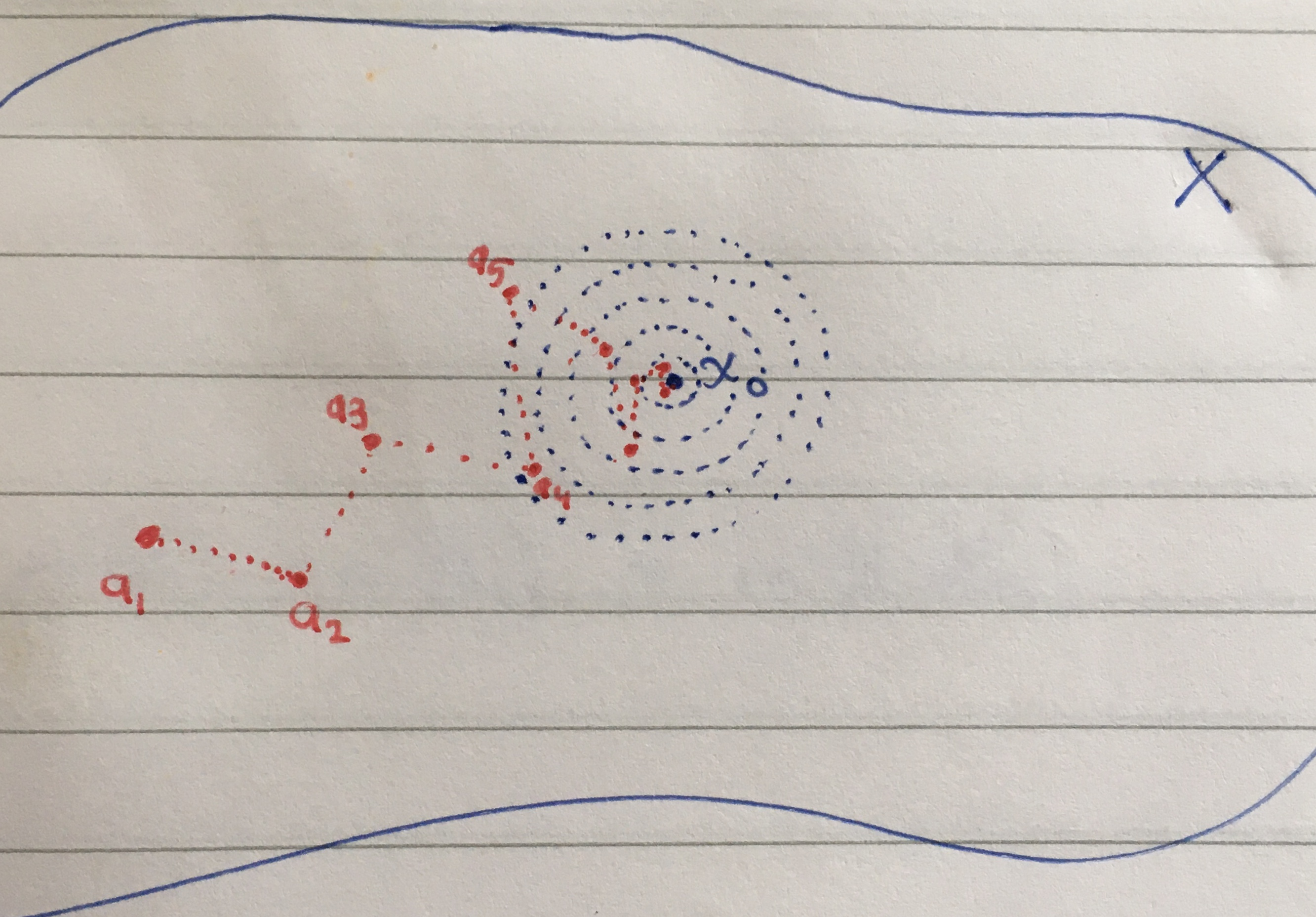Math and science::Topology

# Metric space. Sequence convergence

### Sequence convergence, definition

Let $$X$$ be a metric space, let $$x_0 \in X$$ and let $$(a_n)_{n=1}^{\infty}$$ be a sequence in $$X$$.

$$(a_n)_{n=1}^{\infty}$$ is said to converge to $$x_0$$ iff

[ $$\lim_{?} ? = ?$$ ]

[unfolding the definition of limits, this becomes...]This next result should be internalized

### Lemma. Closed iff [some property of sequences...]

Let $$X$$ be a metric space and $$V \subseteq X$$.

Then $$V$$ is closed in $$X$$ $$\iff$$ [some property of sequences...]

The proof was not immediately obvious to me. I did, however, find a way to visualize it, which I think makes the line of reasoning clear. Part of why the proof is important, I think, is that there are only two notions available from which the result needs to be derived: the definition of open sets and the definition of sequence convergence. So, it should be simple, yet it escapes an immediately obvious proof. Thus, I feel there is a mode of thinking that has its essence somewhat distilled into the line of reasoning.